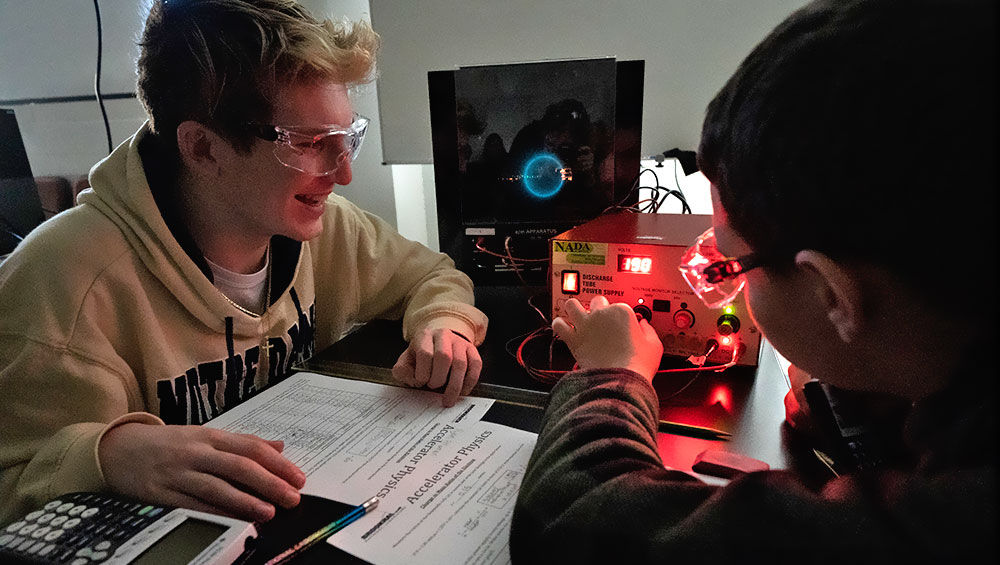General Lab Information

Share

# Accelerator ScienceBrookhaven National Lab's Relativistic Heavy Ion Collider (RHIC) uses electric and magnetic fields to accelerate charged particles in a circle to understand the fundamental building blocks of matter. In this session, students will virtually use our table-top particle accelerators with the help of BNL staff, and then they will graph their data to find the line of best fit and calculate the charge to mass ratio of the electron.

Vocabulary: acceleration, attract, charge, electron, energy, ion, magnetism, repel, speed

Session Information:

• Flexible instruction whether students are in the classroom or at home.
• Interactive, instructor-led session facilitated with students using materials from school or home.  Can also be demonstration-based if students do not have access to materials.
• Teachers will receive a detailed Teacher Notes guide upon booking.
• Secure link to ZoomGov videoconferencing platform will be provided.
• \$300 (BOCES-aidable)
• Time: 1 hour

### New York State Science Standards

AP Physics 1 and 2

Physics 1 Essential Knowledge

Physics 2 Essential Knowledge

1B.3 The smallest observed unit of charge that can be isolated is the electron charge, also known as the elementary charge.

3.C.2 Electric force results from the interaction of one object that has an electric charge with another object that has an electric charge.

4.C.1 The energy of a system includes its kinetic energy, potential energy, and microscopic internal energy. Examples include gravitational potential energy, elastic potential energy, and kinetic energy.

5.A.2 For all systems in all circumstances, energy, charge, linear momentum, and angular momentum are conserved. For an isolated or a closed system, conserved quantities are constant. An open system is one that exchanges any conserved quantity with its surroundings.

1.A.2 Fundamental particles have no internal structure.

1.A.3 Nuclei have internal structures that determine their properties.

1B.3 The smallest observed unit of charge that can be isolated is the electron charge, also known as the elementary charge.

2.C.5 Between two oppositely charged parallel plates with uniformly distributed electric charge, at points far from the edges of the plates, the electric field is perpendicular to the plates and is constant in both magnitude and direction.

2.D.1 The magnetic field exerts a force on a moving electrically charged object. That magnetic force is perpendicular to the direction of velocity of the object and to the magnetic field and is proportional to the magnitude of the charge, the magnitude of the velocity, and the magnitude of the magnetic field. It also depends on the angle between the velocity for angles of 0°, 90°, or 180° and qualitative for other angles.

3.C.2 Electric force results from the interaction of one object that has an electric charge with another object that has an electric charge.

3.C.3 A magnetic force results from the interaction of a moving charged object or a magnet with other moving charged objects or another magnet.

Regents Physics: Core Curriculum

 Process Skills Major Understandings Standard 1, M1.1 Use algebraic and geometric representations to describe and compare data. Standard 1, M2.1 Use deductive reasoning to construct and evaluate conjectures and arguments, recognizing that patterns and relationships in mathematics assist them in arriving at these conjectures and arguments. Standard 1, M3.1 Apply algebraic and geometric concepts and skills to the solution of problems. Standard 1, S3.2 Apply statistical analysis techniques when appropriate to test if chance alone explains the result. Standard 2, 1.1 Understand and use the more advanced features of word processing, spreadsheets, and database software 4.1k Moving electric charges produce magnetic fields. The relative motion between a conductor and a magnetic field may produce a potential difference in the conductor. 5.1j When the net force on a system is zero, the system is in equilibrium. 5.1n Centripetal force* is the net force which produces centripetal acceleration.* In uniform circular motion, the centripetal force is perpendicular to the tangential velocity. 5.1t Gravitational forces are only attractive, whereas electrical and magnetic forces can be attractive or repulsive. 5.3c On the atomic level, energy is emitted or absorbed in discrete packets called photons.*

### Teacher Information

These programs are registered with Eastern Suffolk BOCES